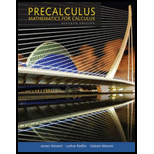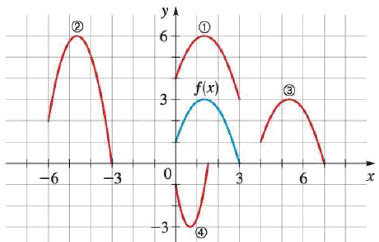Chapter 2.6, Problem 69E### Precalculus: Mathematics for Calcu...

7th Edition
James Stewart + 2 others
ISBN: 9781305071759

#### Solutions

Chapter
Section### Precalculus: Mathematics for Calcu...

7th Edition
James Stewart + 2 others
ISBN: 9781305071759
Textbook Problem

# Identifying Transformations The graph of y = f(x) is given. Match each equation with its graph.69. (a) y = f(x­ − 4) (b) y = f(x) + 3 (c) y = 2f(x + 6) (d) y = −f(2x)(a)

To determine

To identify: The graph of the transformation y=f(x4) from the given graphs.

Explanation

Formula used:

Horizontal shift of graph is, y=f(x+k).

If k>0, shift the graph of f(x) horizontally to the left by k units.

If k<0, shift the graph of f(x) horizontally to the right by k units.

Calculation:

The given function is, y=f(x4)

(b)

To determine

To identify: The graph of the transformation y=f(x)+3 from the given graphs.

(c)

To determine

To identify: The graph of the transformation y=2f(x+6) from the given graphs.

(d)

To determine

To identify: The graph of the transformation y=f(2x) from the given graphs.

### Still sussing out bartleby?

Check out a sample textbook solution.

See a sample solution

#### The Solution to Your Study Problems

Bartleby provides explanations to thousands of textbook problems written by our experts, many with advanced degrees!

Get Started

## Additional Math Solutions

#### Evaluate the integral, if it exists. csc2x1+cotxdx

Single Variable Calculus: Early Transcendentals, Volume I

#### The radius of convergence of is: 1 3 ∞

Study Guide for Stewart's Multivariable Calculus, 8th

#### ln |2x + 1| + C 2 ln |2x + 1| + C

Study Guide for Stewart's Single Variable Calculus: Early Transcendentals, 8th